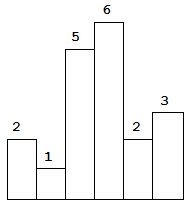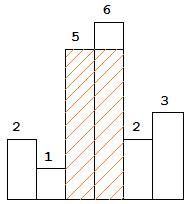# LeetCode 90. Subsets II

## Description

Subsets II

Given a collection of integers that might contain duplicates, nums, return all possible subsets (the power set).

Note: The solution set must not contain duplicate subsets.

Example:

``````Input: [1,2,2]
Output:
[
,
,
[1,2,2],
[2,2],
[1,2],
[]
]
``````

# LeetCode 88. Merge Sorted Array

## Description

Merge Sorted Array

Given two sorted integer arrays nums1 and nums2, merge nums2 into nums1 as one sorted array.

Note:

• The number of elements initialized in nums1 and nums2 are m and n respectively.

• You may assume that nums1 has enough space (size that is greater or equal to m + n) to hold additional elements from nums2.

Example:

``````Input:
nums1 = [1,2,3,0,0,0], m = 3
nums2 = [2,5,6],       n = 3

Output: [1,2,2,3,5,6]
``````

`nums1``nums2`两个有序数组合并到`nums1`一个中，`nums1`已分配足够空间存放元素。

# LeetCode 85. Maximal Rectangle

## Description

Maximal Rectangle

Given a 2D binary matrix filled with 0’s and 1’s, find the largest rectangle containing only 1’s and return its area.

Example:

``````Input:
[
["1","0","1","0","0"],
["1","0","1","1","1"],
["1","1","1","1","1"],
["1","0","0","1","0"]
]
Output: 6
``````

# LeetCode 84. Largest Rectangle in Histogram

## Description

Largest Rectangle in Histogram

Given n non-negative integers representing the histogram’s bar height where the width of each bar is 1, find the area of largest rectangle in the histogram.Above is a histogram where width of each bar is `1`, given height = `[2,1,5,6,2,3]`.The largest rectangle is shown in the shaded area, which has area = `10` unit.

# LeetCode 81. Search in Rotated Sorted Array II

## Description

Search in Rotated Sorted Array II

Suppose an array sorted in ascending order is rotated at some pivot unknown to you beforehand.

(i.e., `[0,0,1,2,2,5,6]` might become `[2,5,6,0,0,1,2]`).

You are given a target value to search. If found in the array return `true`, otherwise return `false`.

Example 1:

``````Input: nums = [2,5,6,0,0,1,2], target = 0
Output: true
``````

Example 2:

``````Input: nums = [2,5,6,0,0,1,2], target = 3
Output: false
``````

Follow up:

• This is a follow up problem to Search in Rotated Sorted Array, where nums may contain duplicates.
• Would this affect the run-time complexity? How and why?

# LeetCode 80. Remove Duplicates from Sorted Array II

## Description

Remove Duplicates from Sorted Array II

Given a sorted array nums, remove the duplicates in-place such that duplicates appeared at most twice and return the new length.

Do not allocate extra space for another array, you must do this by modifying the input array in-place with O(1) extra memory.

Example 1:

``````Given nums = [1,1,1,2,2,3],

Your function should return length = 5, with the first five elements
of nums being 1, 1, 2, 2 and 3 respectively.

It doesn't matter what you leave beyond the returned length.
``````

Example 2:

``````Given nums = [0,0,1,1,1,1,2,3,3],

Your function should return length = 7, with the first seven elements
of nums being modified to 0, 0, 1, 1, 2, 3 and 3 respectively.

It doesn't matter what values are set beyond the returned length.
``````

# LeetCode 79. Word Search

## Description

Word Search

Given a 2D board and a word, find if the word exists in the grid.

The word can be constructed from letters of sequentially adjacent cell, where “adjacent” cells are those horizontally or vertically neighboring. The same letter cell may not be used more than once.

Example:

``````board =
[
['A','B','C','E'],
['S','F','C','S'],
['A','D','E','E']
]

Given word = "ABCCED", return true.
Given word = "SEE", return true.
Given word = "ABCB", return false.
``````

`board`中查找是否存在相连（相邻）的字符与给定的单词对应。

# LeetCode 78. Subsets

## Description

Subsets

Given a set of distinct integers, nums, return all possible subsets (the power set).

Note: The solution set must not contain duplicate subsets.

Example:

Input: nums = [1,2,3] Output: [ , , , [1,2,3], [1,3], [2,3], [1,2], [] ]Programer

ChengDu·China
-